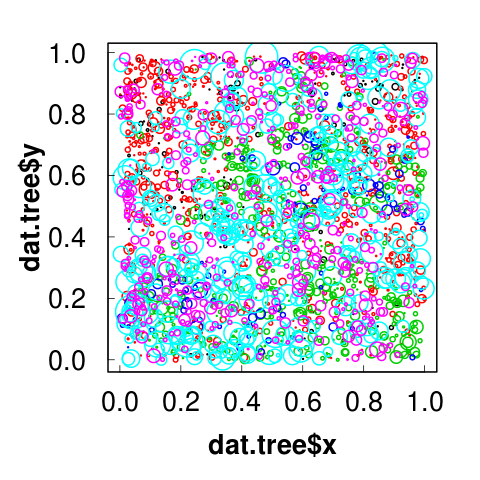# Problem: How to calculate mean tree DBH per species?# Problem: How to calculate mean tree DBH per species?

``````head(dat_tree)

#      x     y  species  dbh status
# 1 0.078 0.091 blackoak 0.85      1
# 2 0.076 0.266 blackoak 0.90      1
# 3 0.051 0.225 blackoak 1.11      1
# 4 0.015 0.366 blackoak 0.18      0
# 5 0.030 0.426 blackoak 0.32      1
# 6 0.102 0.474 blackoak 0.11      1
``````
``````str(dat_tree)
# 'data.frame':	2251 obs. of  5 variables:
#  \$ x      : num  0.078 0.076 0.051 0.015 0.03 0.102 ...
#  \$ y      : num  0.091 0.266 0.225 0.366 0.426 0.474 ...
#  \$ species : Factor w/ 6 levels "blackoak","hickory",..: 1 ...
#  \$ dbh    : num  0.85 0.9 1.11 0.18 0.32 ...
#  \$ status  : int  1 1 1 0 1 1 1 0 1 1 ...
``````# Solution 1: pull out data for each species at a time

• tedious and time-consuming
• high chance of errors
``````# subset out blackoak
bo <-  dat_tree[dat_tree\$species == "blackoak", ]

# x     y  species  dbh status
# 1   0.078 0.091 blackoak 0.85      1
# 2   0.076 0.266 blackoak 0.90      1
# 3   0.051 0.225 blackoak 1.11      1
# 4   0.015 0.366 blackoak 0.18      0
# 5   0.030 0.426 blackoak 0.32      1
# 6   0.102 0.474 blackoak 0.11      1

mean(bo\$dbh)
#  1.258519
``````

# Solution 2: run a loop

• time-consuming and tedious
• lower chance of errors

# Split-apply-combine is a common data analysis pattern

Split: Break a big problem into manageable pieces

Apply: operate on each piece independently

Combine: stick pieces back together

# Examples of split-apply-combine

## Data preparation

• group-wise ranking
• standardization
• normalization
• creating new variables on a per-group basis

## Summaries for display or analysis

• checking sample sizes of groups
• calculating marginal means
• conditioning table of counts by groups

## Modelling

• fitting separate models for groups (=panel)

# Equivalents in other software

Excel pivot tables

APL’s array operators

SQL ‘group by operator’

# apply()

When you want to apply a function to the rows or columns of a matrix (and higher-dimensional analogues).

``````# Two dimensional matrix
M <- matrix(seq(1,16), 4, 4)

M
[,1] [,2] [,3] [,4]
[1,]    1    5    9   13
[2,]    2    6   10   14
[3,]    3    7   11   15
[4,]    4    8   12   16

# apply min to rows
apply(M, 1, min)
 1 2 3 4

# apply max to columns
apply(M, 2, max)
  4  8 12 16
``````

Not generally advisable for data frames as it will coerce to a matrix first.

If you want row/column means or sums for a 2D matrix, look at highly optimized, lightning-quick `colMeans()`, `rowMeans()`, `colSums()`, `rowSums()`

# lapply()

When you want to apply a function to each element of a list in turn and get a list back.

``````x <- list(a = 1, b = 1:3, c = 10:100)

x
\$a
 1

\$b
 1 2 3

\$c
  10  11  12  13  14  15  ... 100

``````
``````lapply(x, FUN = length)
\$a
 1
\$b
 3
\$c
 91

lapply(x, FUN = sum)
\$a
 1
\$b
 6
\$c
 5005
``````

# sapply()

When you want to apply a function to each element of a list in turn, but you want a vector back, rather than a list.

``````x <- list(a = 1, b = 1:3, c = 10:100)

# Compare with above; a named vector, not a list
sapply(x, FUN = length)
a  b  c
1  3 91

sapply(x, FUN = sum)
a    b    c
1    6 5005
``````
``````# lapply:
lapply(x, FUN = sum)
\$a
 1
\$b
 6
\$c
 5005

# sapply:

sapply(x, FUN = sum)
a    b    c
1    6 5005
``````

# tapply()

For when you want to apply a function to subsets of a vector and the subsets are defined by some other vector, usually a factor

``````z <- data.frame(x = 1:20,
y = factor(rep(letters[1:5], each = 4))
)

z
x y
1   1 a
2   2 a
3   3 a
4   4 a
5   5 b
6   6 b
7   7 b
8   8 b
9   9 c
10 10 c
11 11 c
12 12 c
13 13 d
14 14 d
15 15 d
16 16 d
17 17 e
18 18 e
19 19 e
20 20 e
``````
``````# Add up the values of x
#   within each subgroup defined by y:

tapply(z\$x, z\$y, sum)
a  b  c  d  e
10 26 42 58 74
``````

# Functional Programming

R is a functional programming language.

It provides many tools for the creation and manipulation of functions

Anything you can do with vectors, you can do with functions:

• assign them to variables,
• store them in lists,
• pass them as arguments to other functions,
• create them inside functions,
• return them as the result of a function.

# Example: Replacing ‘-99’ with ‘NA’

1. Find and replace in Excel. yikes!

2. Hard code equivalent in R: `df\$a[df\$a == -99] <- NA`
• For every missing value…?
• What if value changes?
3. Functional programming:
• Create function that replaces ‘-99’ with ‘NA’.
• Apply that function to each column.

# Advantages of functional programming this example

Code is more compact.

Can update missing value easily, and in one place.

Code works for any number of columns.

Cannot accidentally miss columns.

Cannot treat one column differently to another.

Can generalise even further.